##### GRE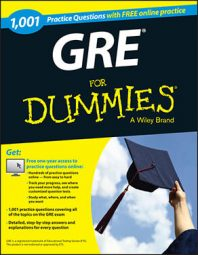On the GRE Math test, Quantitative Comparison problems cover a wide range of subjects. For example, a table or graph question might require you to compare median, range, and standard deviation.

In a Quantitative Comparison question, the problem lists Quantity A and Quantity B, which can be numbers, variables, equations, words, figures, and so on. Your job is to compare these two quantities and determine whether one is greater, they're equal, or the relationship can't be determined.

The following practice questions ask you to compare percentage changes between annual sales for a magazine, and the mean price and standard deviation of the price for a mobile phone.

## Practice questions

1. The graph shows the sales figures for a certain company in five consecutive years.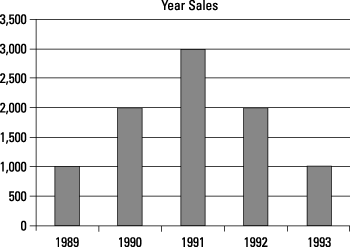Which quantity is greater?

A: The percentage increase in sales from 1989 to 1991

B: The percentage fall in sales from 1991 to 1993

A. Quantity A is greater.

B. Quantity B is greater.

C. The quantities are equal.

D. It cannot be determined from the information given.

2. According to the following table from Forbes magazine, which quantity is greater?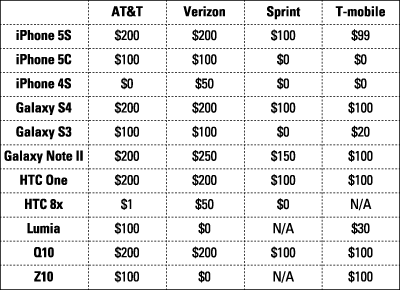A: The mean price of an iPhone 5S

B: The standard deviation of the price of an iPhone 5S

A. Quantity A is greater.

B. Quantity B is greater.

C. The quantities are equal.

D. It cannot be determined from the information given.

1. A. Quantity A is greater.

From 1989 to 1991, the rise is 2,000. From 1991 to 1993, the fall is 2,000.

The percent increase is: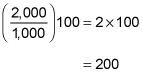The percent fall is: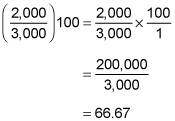Therefore, the percent rise is greater. Choice (A) is the correct answer.

2. A. Quantity A is greater.

To estimate the standard deviation, use the mean deviation. First calculate the mean; then find the average of the distances of each value from the mean: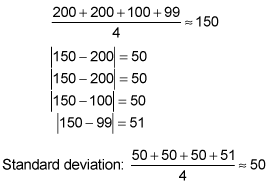Clearly, the mean, Quantity A, is significantly greater, so Choice (A) is the correct answer.